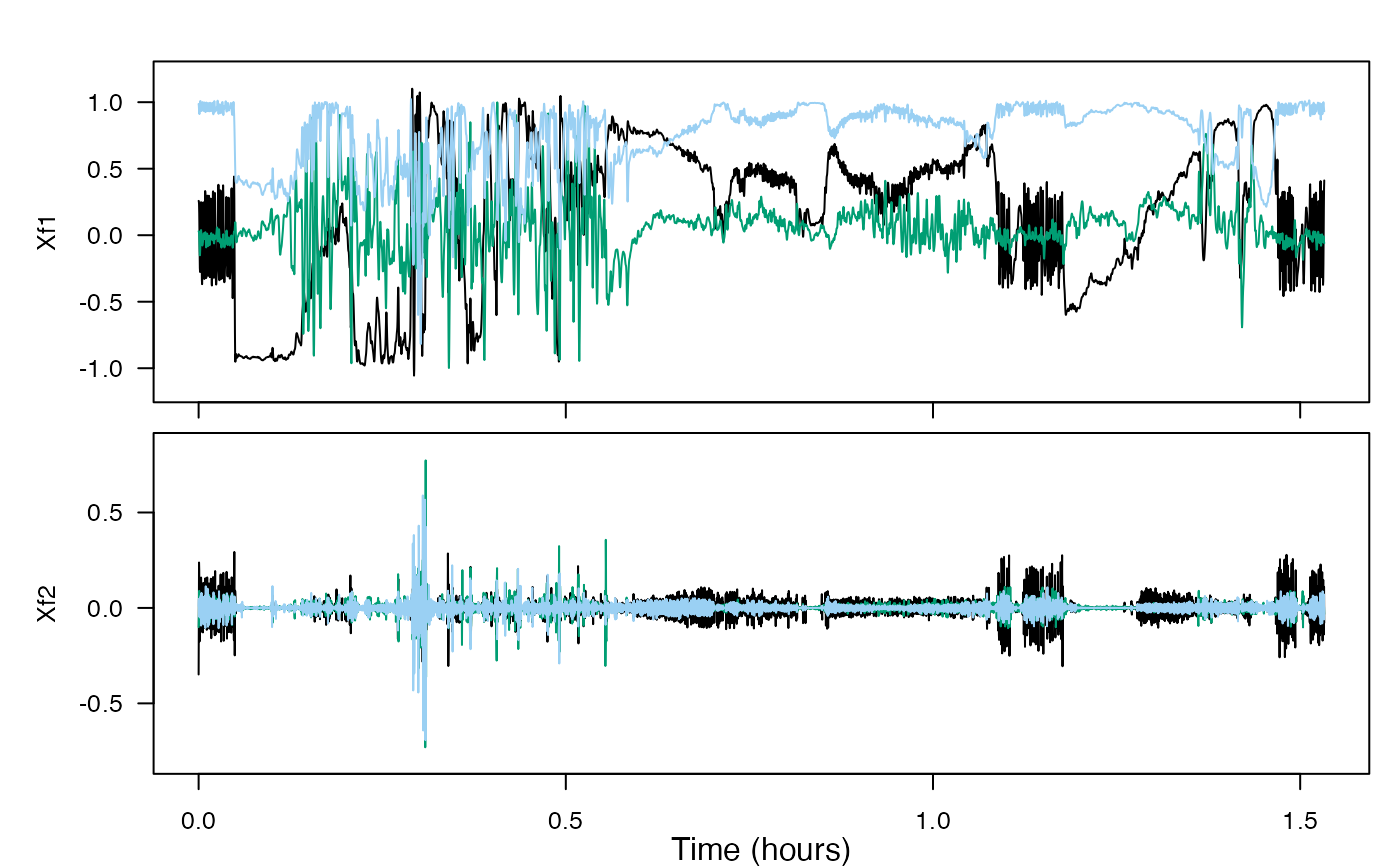This function breaks signal X into two or more frequency bands such that the sum of the signals in the separate bands is equal to the original signal.

## Usage

comp_filt(X, sampling_rate = NULL, fc)

## Arguments

X

A sensor vector or matrix (i.e., with a signal in each column) or sensor list (e.g., from readtag.R).

sampling_rate

The sampling rate of the sensor data in Hz (samples per second).

fc

Specifies the cut-off frequency or frequencies of the complementary filters. Frequencies are in Hz. If one frequency is given, X will be split into a low- and a high-frequency component. If fc contains more than one value, X will be split into multiple complementary bands. Each filter length is 4*sampling_rate/fc. Filtering adds no group delay.

## Value

A list of filtered signals. There are n+1 sections of the list where n is the length of fc. List sections are ordered in Xf from lowest to highest frequency. Each list section contains a vector or matrix of the same size as X, and at the same sampling rate as X.

## Details

Possible input combinations: comp_filt(X,sampling_rate,fc) if X is a vector or matrix, comp_filt(X,fc = fc) if X is a list

## Examples

Xf <- comp_filt(X = beaked_whale$A$data, sampling_rate = beaked_whale$A$sampling_rate, fc = .15)
xf <- list(Xf1 = Xf[], Xf2 = Xf[])
plott(xf, beaked_whale$A$sampling_rate)